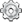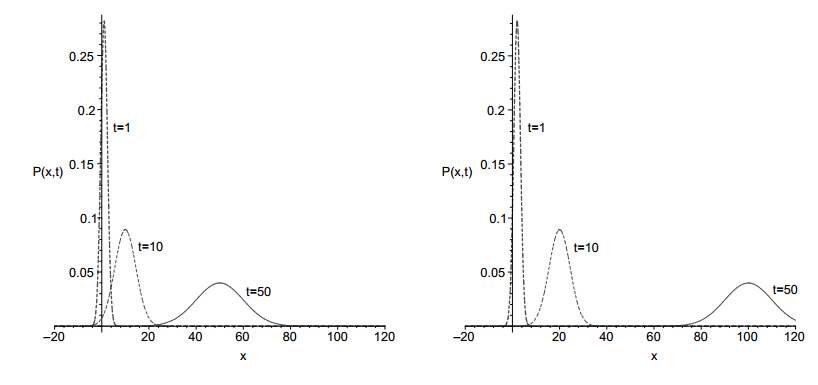# Team:Valencia Biocampus/Demonstration/Diffusion3

(diff) ← Older revision | Latest revision (diff) | Newer revision → (diff)## Proof of a Group Behavior Diffusion Model from a Random Walk Model

To prove that, we will make the assumption that the worm only moves in one dimension ($x$) without loss of generality.

At each time step $\;q\;$ it either moves a distance $\;h\;$ to the left with probability $\;l\;$, a distance $\;h\;$ to the right with probability $\;r\;$, or stays in the same position with probability $\;1−r−l\;$ (the isotropic random walk has $\;r\;=\;l\;=\;1/2$, so it cannot rest motionless). We also deﬁne the probability that a worm is at a position $\;x\;$ at time $\;t\;$ by $\;P(x,t)\;$. One time step earlier, at time $\;t − q\;$, the walker must have been at position $\;x − δ\;$ and then moved to the right, or at position $\;x + δ\;$ and then moved to the left, or at position $\;x\;$ and then not moved at all. Thus:

$$P(x,t)\;=\;P(x,t-q)\;\left(1 - l - q\right) + P(x-h,t-q)\;r + P(x+h,t-q)\;l$$
Assuming that $\;q\;$ and $\;h\;$ are so small, that are negligible compared to $\;t\;$ and $\;x\;$ respectively, we can expand de function as a Taylor series, around $\;t\;$ and $\;x\;$. Notice that higher terms than $\;q^2\;$ and than $\;h^3\;$ have been included in $\;O(q^2)\;$ and $\;O(h^3)\;$, respectively:

$$P\;=\;\left(P - q\;\frac{\partial P}{\partial t}\right)\;\left(1 - l - r\right) + \left(P - q\;\frac{\partial P}{\partial t} - h\;\frac{\partial P}{\partial x} + \frac{h^2}{2}\;\frac{\partial^2 P}{\partial x^2}\right)\;r + \left(P - q\;\frac{\partial P}{\partial t} + h\;\frac{\partial P}{\partial x} + \frac{h^2}{2}\;\frac{\partial^2 P}{\partial x^2}\right)\;l + O(h^3) + O(q^2)$$
Rearranging this gives:

$$\frac{\partial P}{\partial t}\;=\;\frac{\alpha\;h^2}{2\;q}\;\frac{\partial^2 P}{\partial x^2} - \frac{\beta\;h}{q}\;\frac{\partial P}{\partial x} + O(h^3) + O(q^2)$$
Where $\;\alpha\;=\;r + l\;$ and $\;\beta\;=\;r - l\;$. We now let $\;h,\;q,\;\beta\;\rightarrow\;0\;$ in such a way that the following limits are ﬁnite:

$$D\;=\;\alpha\;\lim\limits_{h,\;q,\;\beta\rightarrow 0}\frac{h^2}{2\;q}$$ $$v\;=\;\lim\limits_{h,\;q,\;\beta\rightarrow 0}\frac{h\;\beta}{q}$$
So we can neglect $\;O(h^3)\;$ and $\;O(q^2)\;$, resting:

$$\frac{\partial P}{\partial t}\;=\;D\;\frac{\partial^2 P}{\partial x^2} - v\;\frac{\partial P}{\partial x}$$
Considerations:

• If we set $\;r\;=\;l\;=\;1/2\;$ as in the isotropic random walk, then $\;\beta\;=\;0\;$, so $\;u\;=\;0\;$, giving as a result the non-biased Diffusion Equation:

$$\frac{\partial P}{\partial t}\;=\;D\;\frac{\partial^2 P}{\partial x^2}$$
• In this case, $\;v\;$ is constant for all the space, not as in the case that concerns us, where $\;v\;$ depends on the gradient of the attractant, normally distributed (with Gaussian Distributions) in the space of interest. So, with $\;v\;$ constant, it is possible to obtain an analytical solution, given by Montroll & Shlesinger (1984), with initial condition $\;P(x,0)\;=\;\delta(x)\;$, that is:

$$P(x,t)\;=\;\frac{1}{\sqrt{4 \pi D t}}\;e^{-\left(x - v t\right)^2/\left(4 D t\right)}$$Plots of $\;P(x,t)\;$ for different $\;v\;$, and different time instants: left, $\;D$ = $1\;$ and $\;v$ = $1\;$; right, $\;D$ = $1\;$ and $\;v$ = $2\;$

Okopinska A. (2002) Fokker-Planck equation for bistable potential in the optimized expansion. Physical review E, Volume 65, 062101